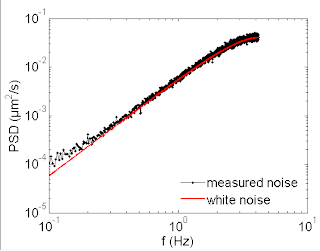## Saturday, April 12, 2008

### [MT 3] Differential Discrete White Noise

Assume a white noise discrete time series (sampled at intervalls of dt) with variance sigma^2, here interpreted as a fluctuating position variable:

The discrete velocity is defined as:

Its autocorrelation yields (using the Cxx(m) above):

The PSD of the velocity fluctuations is given by the Fourier transform of Cvv. We have to use the Discrete Fourier Transform (DFT) here:

The DFT is based on discrete times

and the corresponding frequencies

where the indices run as follows:

One obtains the following PSD of the velocity fluctuations:In a log-log plot, this looks as shown in this figure. The slope is always 2. So in the case of the experimental bead PSD, the apparent fractional slope of the high frequency wing comes from the superposition with the low frequency fractional powerlaw.

For a detailed derivation of the above formulae, see
SharedDocuments/Notes/DifferentialDiscreteWhiteNoise.pdf

The following figure shows a comparison between the above theoretical PSD and experimental data, measured for a purely noisy bead with a flat MSD: